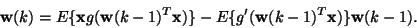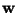Next: Tensor-based algorithms Up: Algorithms for ICA Previous: Other neural (adaptive) algorithms

## The FastICA algorithm

Adaptive algorithms based on stochastic gradient descent may be problematic when used in an environment where no adaptation is needed. This is the case in many practical situations. The convergence is often slow, and depends crucially on the choice of the learning rate sequence. As a remedy for this problem, one can use batch (block) algorithms based on fixed-point iteration [72,60,65]. In , a fixed-point algorithm, named FastICA, was introduced using kurtosis, and in [60,65], the FastICA algorithm was generalized for general contrast functions. For sphered data, the one-unit FastICA algorithm has the following form:(41)

where the weight vectoris also normalized to unit norm after every iteration, and the function g is the derivative of the function G used in the general contrast function in Eq. (28). The expectations are estimated, in practice, using sample averages over a sufficiently large sample of the input data. Units using this FastICA algorithm can then be combined, just as in the case of neural learning rules, into systems that estimate several independent components. Such systems may either estimate the independent component one-by-one using hierarchical decorrelation (deflation), or they may estimate all the independent components in parallel, with symmetric decorrelation [72,65]. Versions of (41) that need no preliminary sphering were also introduced in .

The FastICA algorithm is neural in that it is parallel and distributed, but it is not adaptive. Instead of using every data point immediately for learning, FastICA uses sample averages computed over larger samples of the data. The convergence speed of the fixed-point algorithms is clearly superior to those of the more neural algorithms. Speed-up factors in the range from 10 to 100 are usually observed .

An interesting point proven in  is that when FastICA is used with symmetric decorrelation, it is essentially equivalent to a Newton method for maximum likelihood estimation. This means that FastICA is a general algorithm that can be used to optimize both one-unit and multi-unit contrast functions.Next: Tensor-based algorithms Up: Algorithms for ICA Previous: Other neural (adaptive) algorithms
Aapo Hyvarinen
1999-04-23